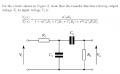# RC bandpass filter transfer function derivation

Status
Not open for further replies.

#### Mitchy190

Joined Apr 23, 2012
33
Hey, I am having some difficulty with deriving the transfer function of the circuit below:From learning Laplace, I gathered you could work out the high and low pass transfer functions separately by converting the circuit into the s-domain, and then multiplying them together to get the overall function. But I now know this is invalid with this circuit, due to the loading effects.

So my question is, how do I go about deriving the transfer function now?

Your help is much appreciated!Thanks

#### The Electrician

Joined Oct 9, 2007
2,916
I see that you posted this same question on another forum.

This problem is simple enough that you don't need to use any of the advanced network analysis techniques, such as node or mesh analysis.

You can treat the network as a cascade of voltage dividers.

Calculate the equivalent impedance of R2 and C2 in series. Call that impedance Z1. Now calculate the equivalent impedance of Z1 in parallel with C1; call that impedance Z2.

Now R1 and Z2 form a voltage divider and you can calculate the voltage at the top of C1 as a fraction (call that fraction tf1) of Vi; call that voltage Vx.

Now, C2 and R2 form a voltage divider acting on Vx. You can calculate Vo as a fraction of Vx (call that fraction tf2).

Having the voltage divider ratio between Vx and Vi (tf1 = Vx/Vi), and the ratio between Vo and Vx (tf2 = Vo/Vx), you can calculate the ratio between Vo and Vi as the product of tf1 and tf2. Your desired transfer function is Vo/Vi. We know that Vo/Vi = (Vx/Vi)*(Vo/Vx) = tf1*tf2; problem solved.

The reason this product of separate "transfer functions" (what I called tf1 and tf2) works, whereas the product of the separate high and low pass transfer functions doesn't give the correct answer, is that tf1 was calculated taking into account the loading effect of the following high pass section.

Give it a try and post your results here.

•Mitchy190

#### Mitchy190

Joined Apr 23, 2012
33
Thank for your reply! Ive just started doing it, I will post my results#### Mitchy190

Joined Apr 23, 2012
33

#### automagp68

Joined Nov 13, 2011
81
How exactly are you using a voltage divider here

This is not going to give you the magnitude of the output but instead the magnitude of the output for an equivalent circuit where the output is defined in a different location.

#### WBahn

Joined Mar 31, 2012
28,186
Since the TS last responded to this thread ~2.5 years ago, it is highly likely that they have resolved their problem or are no longer interested in doing so.

Status
Not open for further replies.